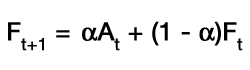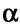### Exponential Smoothing

In this method the next period's forecast is a weighted average of all previous observations that gives progressively less weight to older observations. Forecasts using exponential smoothing are simple to compute; thus, it is a very popular forecasting method that can be made as sensitive as required. This approach is called exponential smoothing because the forecast that is generated is made up of an exponentially weighted average of all previous observations. The averaging techniques discussed earlier are known as "smoothing" processes as they attempt to remove the random fluctuations from the time series so that the underlying trend can be seen more clearly and can thus be used for making a forecast that is not subject to random swings. Exponential smoothing was invented by R. G. Brown in the 1950s to make short term forecasts, primarily for the time period following the latest observation. The exponential smoothing formula is given by:, whereis a smoothing factor, a fraction between 0 and 1.

The weights attached to each observed value in the series of values that make up any

"forecast", Ft+1 form an exponential series with the greatest weight being attached to the most recent observation. The weight for each of the preceding observation decreases exponentially by a fixed fraction (1-).

The sensitivity of the forecast to changes in the most recently observed data is controlled by the factor. Ifis set to 1 the new forecast (smoothed value) will be equal to the latest observation and there will be no smoothing. The implication in this case is that the new forecast should respond immediately to changes in the actual observation seen in the most recent period. On the other hand, ifis set to 0, then all variations in the actual value from the initial forecast is ignored and the new forecast remains the same as the previous forecast value. This implies that the actual value in the most recent period is purely a random occurrence and hence should be ignored. In practice, however, the value chosen foris between 0.1 and 0.3.

In order to initialize exponential smoothing, a forecaster needs two pieces of information--an initial forecast and a value for. The value ofis left to the judgment of the forecaster. An initial forecast can be obtained using the naïve approach by assuming that it is equal to the actual value from the previous period. We will now go through a simple example of generating forecasts using exponential smoothing.